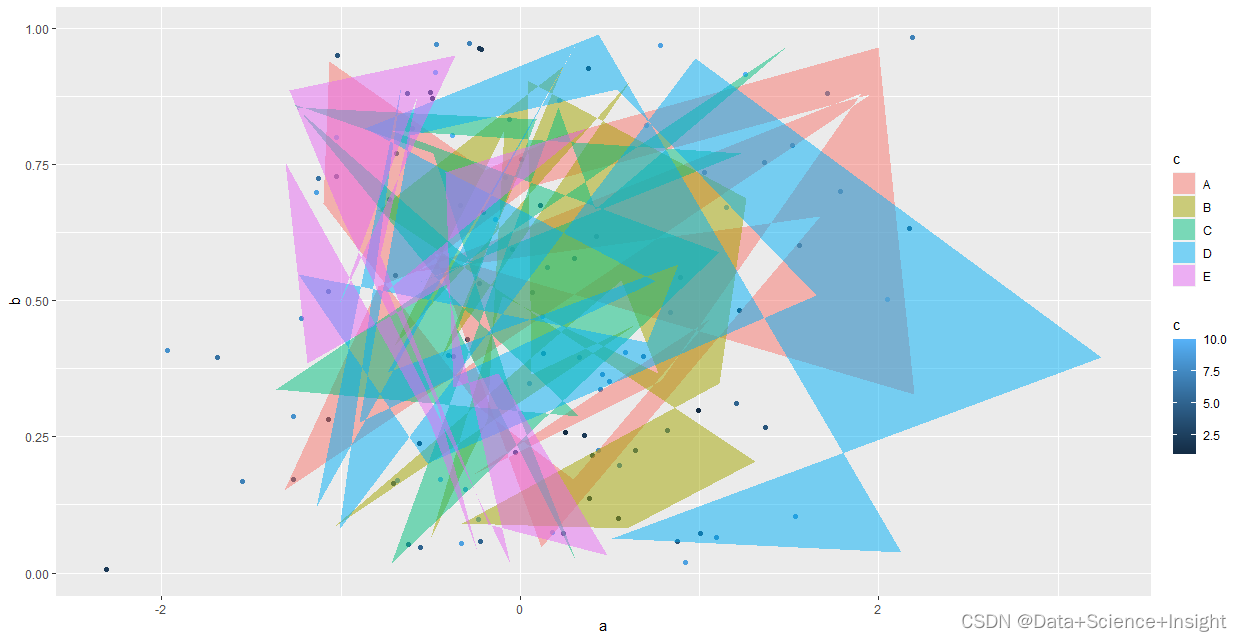# [Solved] R Language Error: Discrete value supplied to continuous scale

Error: discrete value supplied to continuous scale

#Simulation data

``````set.seed(123)
my_df1 <- data.frame(a=rnorm(100), b=runif(100), c=rep(1:10, 10))
my_df2 <- data.frame(a=rnorm(100), b=runif(100), c=factor(rep(LETTERS[1:5], 20)))
``````

#Error: Discrete value supplied to continuous scale

``````ggplot() +
geom_point(data=my_df1, aes(x=a, y=b, color=c)) +
geom_polygon(data=my_df2, aes(x=a, y=b, color=c), alpha=0.5)``````

#Solution:

Use the fill parameter;

``````ggplot() +
geom_point(data=my_df1, aes(x=a, y=b, color=c)) +
geom_polygon(data=my_df2, aes(x=a, y=b, fill=c), alpha=0.5)``````